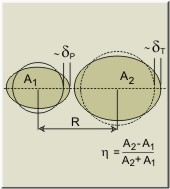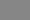Model: sudden approximation (diabatic) adiabatic proximity + parabolic deformation energy folding     + parabolic deformation energy proximity + TCSM deformation energy folding     + TCSM deformation energy liquid drop model (LDM) two-center shell model (TCSM)
 Projectileβ2g.s. Targetβ2g.s.
 Chose one, two, or three variables Input range of variation fixed value minimum maximum number of points Distance between mass centers, R Deformation of projectile, δP Deformation of target, δT Unified deformation (see description), δ Mass asymmetry, η=(A2-A1)/(A2+A1) Orientation of projectile in reaction plane, ΘP Orientation of target in reaction plane, ΘT Azimuthal orientation, φ Neck parameter, ε recommended value:1 (fusion), 0.35 (fission)
 Basic quantities (to be changed by advanced users only)             Default values Folding target r0(p) r0(n) a(p) a(n) amplitudes of Migdal NN-potential fin fin' projectile r0(p) r0(n) a(p) a(n) fex fex' Proximity r0(target) r0(proj) b